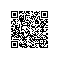# 局部方法$("html").load()和全局方法$.get()、$.post()-阿里云开发者社区 # 局部方法$("html").load()和全局方法$.get()、$.post()

.load()方法可以参数三个参数：url(必须，请求 html 文件的 url 地址，参数类型为 String)、
data(可选，发送的 key/value 数据，参数类型为 Object)、callback(可选，成功或失败的回调

//HTML
<input type="button" value="异步获取数据" />
<div id="box"></div>
//jQuery
$('input').click(function () {$('#box').load('test.html');
});

//带选择器的 url
$('input').click(function () {$('#box').load('test.html .my');
});

//不传递 data，则默认 get 方式
$('input').click(function () {$('#box').load('test.php?url=ycku');
});
//get 方式接受的 PHP
<?php
if ($_GET['url'] == 'ycku') { echo '瓢城 Web 俱乐部官网'; } else { echo '其他网站'; } ?> //传递 data，则为 post 方式$('input').click(function () {
$('#box').load('test.php', { url : 'ycku' }); }); //post 方式接受的 PHP <?php if ($_POST['url'] == 'ycku') {
echo '瓢城 Web 俱乐部官网';
} else {
echo '其他网站';
}
?>

（XMLHttpRequest 对象）。
$('input').click(function () {$('#box').load('test.php', {
url : 'ycku'
}, function (response, status, xhr) {
alert('返回的值为：' + response + '，状态为：' + status + '，

});
});

responseText 作为响应主体被返回的文本
responseXML

status 响应的 HTTP 状态
statusText HTTP 状态的说明

HTTP 状态码 状态字符串 说明
200 OK 服务器成功返回了页面
401 Unauthorized 请求需要用户认证
500 Internal Server Error 服务器遇到意外错误，无法完成请求
503 ServiceUnavailable 由于服务器过载或维护导致无法完成请求

.load()方法是局部方法，因为他需要一个包含元素的 jQuery 对象作为前缀。而$.get()和$.post()是全局方法，无须指定某个元素。对于用途而言，.load()适合做静态文件的异步获取，

$.get()方法有四个参数，前面三个参数和.load()一样，多了一个第四参数 type，即服务 器返回的内容格式：包括 xml、html、script、json、jsonp 和 text。第一个参数为必选参数， 后面三个为可选参数。 //使用$.get()异步返回 html 类型
$('input').click(function () {$.get('test.php', {
url : 'ycku'
}, function (response, status, xhr) {
if (status == 'success') {
$('#box').html(response); } }) //type 自动转为 html }); 注意：第四参数 type 是指定异步返回的类型。一般情况下 type 参数是智能判断，并不 需要我们主动设置，如果主动设置，则会强行按照指定类型格式返回。 //使用$.get()异步返回 xml
$('input').click(function () {$.get('test.xml', function (response, status, xhr) {
$('#box').html($(response).find('root').find('url').text());
}); //type 自动转为 xml
});

//使用$.get()异步返回 json$.get('test.json', function (response, status, xhr) {
});
$.post()方法的使用和$.get()基本上一致，他们之间的区别也比较隐晦，基本都是背后的

1.GET 请求是通过 URL 提交的，而 POST 请求则是 HTTP 消息实体提交的；
2.GET 提交有大小限制（2KB），而 POST 方式不受限制；
3.GET 方式会被缓存下来，可能有安全性问题，而 POST 没有这个问题；
4.GET 方式通过$_GET[]获取，POST 方式通过$_POST[]获取。
//使用$.post()异步返回 html$.post('test.php', {
url : 'ycku'
}, function (response, status, xhr) {
$('#box').html(response); }); 四．$.getScript() 和$.getJSON() jQuery 提供了一组用于特定异步加载的方法：$.getScript()，用于加载特定的 JS 文件；
$.getJSON()，用于专门加载 JSON 文件。 有时我们希望能够特定的情况再加载 JS 文件，而不是一开始把所有 JS 文件都加载了， 这时课时使用$.getScript()方法。
//点击按钮后再加载 JS 文件
$('input').click(function () {$.getScript('test.js');
});
$.getJSON()方法是专门用于加载 JSON 文件的，使用方法和之前的类似。$('input').click(function () {
\$.getJSON('test.json', function (response, status, xhr) {
});
});使用钉钉扫一扫加入圈子
+ 订阅